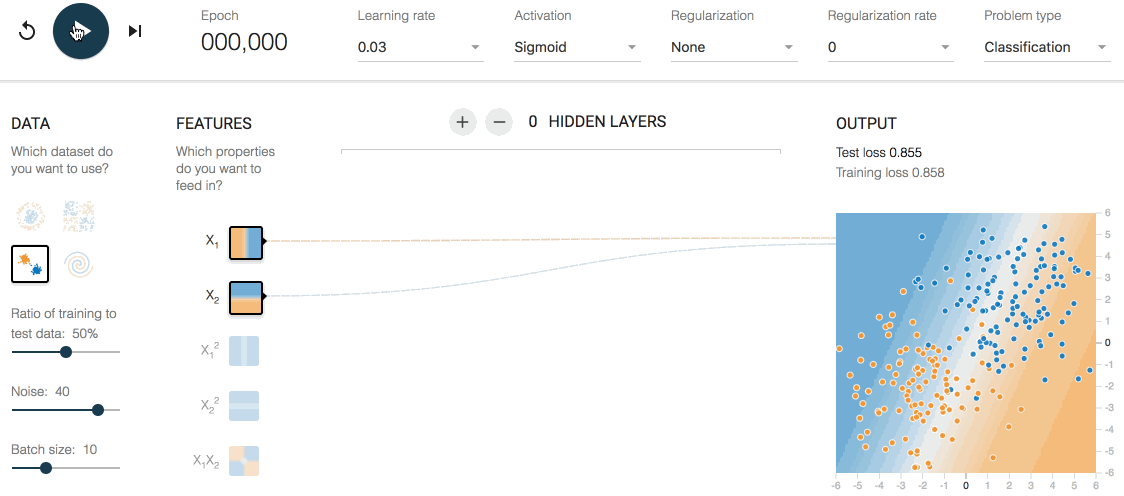## Logistic 回归

$$h_\boldsymbol{\theta}(\boldsymbol{x})=g(\boldsymbol{\theta}^ \mathrm{T}\boldsymbol{x})=\frac{1}{1+e^{-\boldsymbol{\theta}^ \mathrm{T}\boldsymbol{x}}}$$$$g’(z)=\frac{d}{dz}\frac{1}{1+e^{-z}}=\frac{e^{-z}}{(1+e^{-z})^2}=g(z)\left(1-g(z)\right)$$

$z$ 趋于正无穷时，$g(z)$ 趋于 1；$z$ 趋于负无穷时，$g(z)$ 趋于 0。

$h_\boldsymbol{\theta}(\boldsymbol{x}) \geq 0.5$ 即 $\boldsymbol{\theta}^\mathrm{T}\boldsymbol{x}\geq0$ 表示是恶性肿瘤的可能性大于等于 50%，因此可以给定一个阈值例如就是 0.5，恶性肿瘤的可能性大于等于 50% 就判定是恶性肿瘤，否则判定不是恶性肿瘤。即
$$y = \begin{cases} 0 & h_\boldsymbol{\theta}(\boldsymbol{x}) < 0.5 \\ 1 & h_\boldsymbol{\theta}(\boldsymbol{x}) \geq 0.5 \end{cases}$$

$$E_{\theta}=\sum_{i=1}^m(y^{(i)}-\frac{1}{1+e^{-\boldsymbol{\theta}^ \mathrm{T}\boldsymbol{x}^{(i)}}})^2$$

## 两大学派的争论

• 概率：描述了已知参数 $\theta$ 时的随机变量的输出结果。例如已知参数 $\theta=0.5$ ，求抛 m 次硬币出现 n 次正面朝上的概率。
• 似然：描述已知随机变量输出结果时，未知参数 $\theta$ 的可能取值。例如已知抛 100 次硬币出现 50 次正面朝上，求参数 $\theta=x$ 的似然程度。

### 贝叶斯学派

$$P(\theta|X)=\frac{P(X|\theta)P(\theta)}{P(X)}$$

#### 先验概率 $P(\theta)$#### 似然函数 $P(X|\theta)$

$$P(X|\theta)=\binom{10}{2}\theta^2(1-\theta)^8$$### 最大似然估计(MLE)

Maximun Likelihood Estimation 是频率学派广泛用的估计方法。假设数据 $X$是独立同分发布的一组抽样。那么 MLE 对 $\theta$ 的估计方法可以如下推导：
\begin{align} \hat\theta_{MLE} & = \arg \max P(X;\theta) \\ & = \arg \min -log P(X;\theta) \end{align}

### 最大后验估计(MAP)

Maximum A Posteriori 是贝叶斯学派广泛使用的估计方法。假设数据 $X$ 是独立同分发布的一组抽样。那么 MAP 对 $\theta$ 的估计方法可以如下推导：
\begin{align} \hat\theta_{MAP} & = \arg \max P(\theta|X) \\ & = \arg \min - log P(\theta|X) \\ & = \arg \min - log P(X|\theta)-log P(\theta)+log P(X) \\ & = \arg \min - log P(X|\theta)-log P(\theta) \end{align}

MLE 和 MAP 在优化时的不同就是在于先验项 $-log P(\theta)$。假设在某次实验中，先验分布是标准高斯分布，即参数 $\theta$ 满足标准高斯分布，则 $P(\theta) = Ce^{-\frac{\theta^2}{2}}$，$-log P(\theta) = C + \frac{\theta^2}{2}$。所以在 MAP 中选择标准高斯分布作为先验分布时就等价于在 MLE 中采用了 L2 的正则化项。

## 代价函数$$loss\left(h_\boldsymbol{\theta}(\boldsymbol{x}), y\right) = \begin{cases} -log\left(h_\boldsymbol{\theta}(\boldsymbol{x})\right) & y=1 \\ -log\left(1-h_\boldsymbol{\theta}(\boldsymbol{x})\right) & y=0 \end{cases}$$

$$J(\boldsymbol{\theta}) =-\frac{1}{m}\sum_{i=1}^m\Big(y^{(i)}log\left(h_\boldsymbol{\theta}(\boldsymbol{x}^{(i)})\right)+(1-y^{(i)})log\left(1-h_\boldsymbol{\theta}(\boldsymbol{x}^{(i)})\right)\Big)$$

\begin{align} \nabla_{\theta_j}J(\boldsymbol{\theta}) & = \frac{\partial{J(\boldsymbol{\theta})}}{\partial{\theta_j}} \\ & = -\frac{1}{m}\sum_{i=1}^m\left(\frac{y^{(i)}}{h_\boldsymbol{\theta}(\boldsymbol{x}^{(i)})}\frac{\partial{h_\boldsymbol{\theta}(\boldsymbol{x}^{(i)})}}{\partial{\theta_j}}-\frac{1-y^{(i)}}{1-h_\boldsymbol{\theta}(\boldsymbol{x}^{(i)})}\frac{\partial{h_\boldsymbol{\theta}(\boldsymbol{x}^{(i)})}}{\partial{\theta_j}}\right) \\ & = \frac{1}{m}\sum_{i=1}^m\left(h_\boldsymbol{\theta}(\boldsymbol{x}^{(i)})-y^{(i)}\right)x^{(i)}_j \end{align}

## Softmax 回归

Softmax 回归是对率回归的推广，当类别数 K=2 的时候，Softmax 回归退化为对率回归。由于在模型中使用了 Softmax 函数，比较温和(soft)地输出样本属于各个类别的概率，而不是直接最可能属于的类别，因此叫做 Softmax 回归。

Softmax 函数或称归一化指数函数，是 Sigmoid 函数的一种推广。它能将一个含任意实数的 K 维向量 $\boldsymbol{z}$ “压缩”到另一个 K 维实向量 $\sigma(\boldsymbol{z})$ 中，使得每一个元素的范围都在 (0, 1) 之间，并且所有元素的和为 1。该函数的形式通常按下面的式子给出：
$$\sigma(\boldsymbol{z})=\frac{1}{\sum_{i=1}^ke^{z_i}}\begin{bmatrix} e^{z_1} \\ e^{z_2} \\ … \\ e^{z_k} \end{bmatrix}\quad$$
Softmax 回归模型对于诸如 MNIST 手写数字分类等问题很有用。对于二分类问题，类标记 $y\in\lbrace0, 1\rbrace$；而在 K(K > 2) 分类问题中则是 $y\in\lbrace1, 2, …, k\rbrace$。例如，在 MNIST 数字识别任务中，10 个数字对应 K=10 个不同的类别。

$$h_\boldsymbol{\Theta}(\boldsymbol{x})=\begin{bmatrix} p(y=1|\boldsymbol{x};\boldsymbol{\Theta}) \\ p(y=2|\boldsymbol{x};\boldsymbol{\Theta}) \\ … \\ p(y=k|\boldsymbol{x};\boldsymbol{\Theta}) \end{bmatrix}=\frac{1}{\sum_{i=1}^ke^{\boldsymbol{\theta}^\mathrm{T}_i\boldsymbol{x}}}\begin{bmatrix} e^{\boldsymbol{\theta}^\mathrm{T}_1\boldsymbol{x}} \\ e^{\boldsymbol{\theta}^\mathrm{T}_2\boldsymbol{x}} \\ … \\ e^{\boldsymbol{\theta}^\mathrm{T}_k\boldsymbol{x}} \end{bmatrix}\quad 其中 \boldsymbol{\Theta}=\begin{bmatrix} -\boldsymbol{\theta}^\mathrm{T}_1- \\ -\boldsymbol{\theta}^\mathrm{T}_2- \\ … \\ -\boldsymbol{\theta}^\mathrm{T}_k- \end{bmatrix}$$

### 代价函数

$$J(\boldsymbol{\Theta}) =-\frac{1}{m}\sum_{i=1}^m\sum_{j=0}^11\lbrace y^{(i)}=j\rbrace logp(y^{(i)}=j|\boldsymbol{x}^{(i)};\boldsymbol{\Theta})$$

$$J(\boldsymbol{\Theta}) =-\frac{1}{m}\sum_{i=1}^m\sum_{j=1}^k1\lbrace y^{(i)}=j\rbrace log\frac{e^{\boldsymbol{\theta}_j^\mathrm{T}\boldsymbol{x}^{(i)}}}{\sum_{l=1}^ke^{\boldsymbol{\theta}^\mathrm{T}_l\boldsymbol{x}^{(i)}}}$$

\begin{align} \frac{\partial{J(\boldsymbol{\Theta})}}{\partial{\boldsymbol{\theta}_j}} = -\frac{1}{m} \sum_{i=1}^m\left(\boldsymbol{x}^{(i)}(1\lbrace y^{(i)}=j\rbrace-\frac{e^{\boldsymbol{\theta}_j^\mathrm{T}\boldsymbol{x}^{(i)}}}{\sum_{l=1}^ke^{\boldsymbol{\theta}^\mathrm{T}_l\boldsymbol{x}^{(i)}}})\right) \end{align}

## * 神经网络## 参考文献

 周志华. 机器学习. 清华大学出版社. 2016.

 吴恩达. DeepLearning.

 Ian Goodfellow, Yoshua Bengio, Aaron Courville. Deep Learning. 人民邮电出版社. 2017.

 Stephen Boyd, Lieven Vandenberghe. 凸优化. 清华大学出版社. 2017.

 Christopher M.Bishop. Pattern Recognition and Machine Learning. 2006.

0%